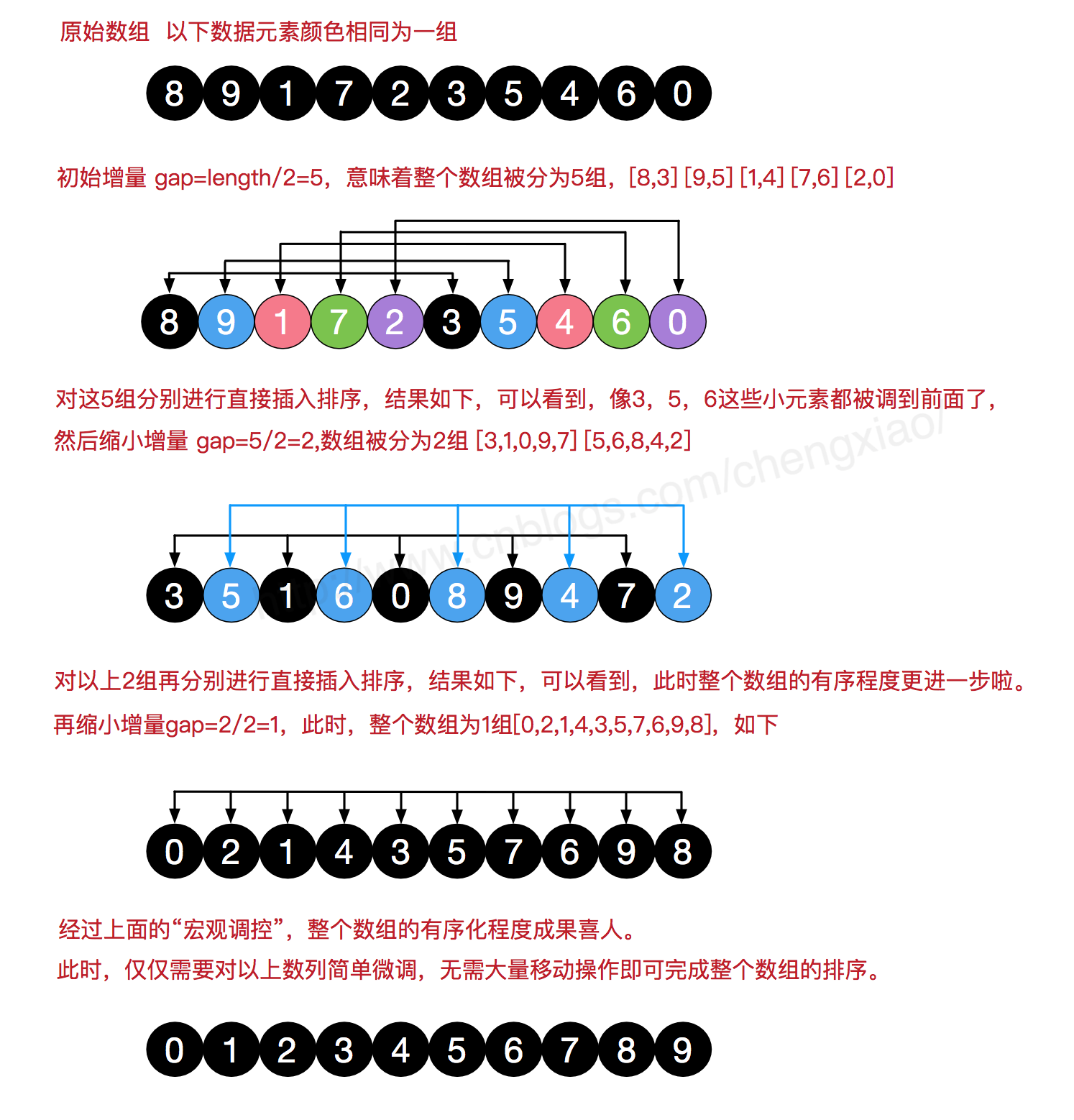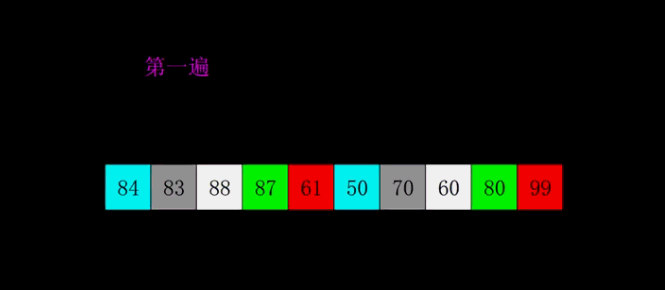﻿ 希尔排序的原理解析

#我们来看下希尔排序的基本步骤，在此我们选择增量gap=length/2，缩小增量继续以gap = gap/2的方式，这种增量选择我们可以用一个序列来表示，{n/2,(n/2)/2...1}，称为增量序列。希尔排序的增量序列的选择与证明是个数学难题，我们选择的这个增量序列是比较常用的，也是希尔建议的增量，称为希尔增量，但其实这个增量序列不是最优的。此处我们做示例使用希尔增量。#在希尔排序的理解时，我们倾向于对于每一个分组，逐组进行处理，但在代码实现中，我们可以不用这么按部就班地处理完一组再调转回来处理下一组（这样还得加个for循环去处理分组）比如[5,4,3,2,1,0] ，首次增量设gap=length/2=3,则为3组[5,2] [4,1] [3,0]，实现时不用循环按组处理，我们可以从第gap个元素开始，逐个跨组处理。同时，在插入数据时，可以采用元素交换法寻找最终位置，也可以采用数组元素移动法寻觅。希尔排序的代码比较简单，如下：

//希尔排序
void shell_sort1(int a[], int n)
{
int i,j,gap;

// gap为步长，每次减为原来的一半。
for (gap = n / 2; gap > 0; gap /= 2)
{
// 共gap个组，对每一组都执行直接插入排序
for (i = 0 ;i < gap; i++)
{
for (j = i + gap; j < n; j += gap)
{
// 如果a[j] < a[j-gap]，则寻找a[j]位置，并将后面数据的位置都后移。
if (a[j] < a[j - gap])
{
int tmp = a[j];
int k = j - gap;
while (k >= 0 && a[k] > tmp)
{
a[k + gap] = a[k];
k -= gap;
}
a[k + gap] = tmp;
}
}
}

}
}

main()
{int a={4,6,3,5,2},i,j,temp;
shell_sort1(a,5);
for(i=0;i<5;i++)
printf("%d ",a[i]);
}